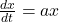## The rate at which a quantity M of a certain radioactive substance decays is proportional to the amount of the substance present at a given t

Question

The rate at which a quantity M of a certain radioactive substance decays is proportional to the amount of the substance present at a given time. Which of the following is a differential equation that could describe this relationship?
1. dM/dt = -3.72 t^2
2. dM/dt = -0.11M
3. dM/dt = 0.08 t^2
4. dM/dt = 1.2M

in progress 0
3 months 2021-08-26T05:23:04+00:00 1 Answers 2 views 0

2. dM/dt = -0.11M

Step-by-step explanation:

Differential equations for proportional amouts:

A differential equation for proportional amounts is given by:In which a is the constant rate. If the amount increases, a is positive. If it decays, b is positive.

In this question:

Decays, so a < 0.

Proportional to the amount of the substance present at a given time, which means that a multiplies the amount M.

This means that the correct answer is given by option 2.